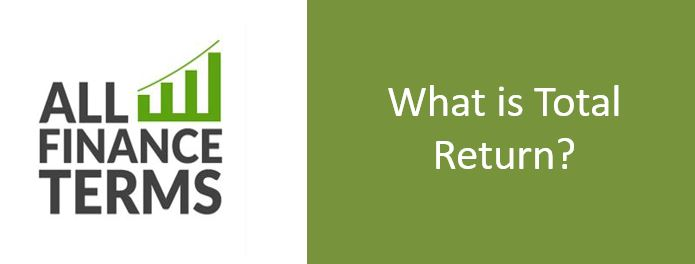## Definition of Total Return

One of the major goals of making investments is to earn profit returns from it. The total return income generally includes capital gains, interests ,distributions and dividends received over the period.

Total Return =Dividends+ interests +distributions+ capital gain- capital loss

Total return can be divided into two categories, regular interest or dividends and capital gains i.e. appreciation in price of investment or asset. The other term is price return which includes only capital gain on investment. Total return is normally expressed in percentage form.

### Brief Explanation of Total Return

let us take an example.

An investor purchases 10 shares of value \$20 per share with initial total value of \$ 200.Share dividend return is 10 % and if the investor reinvests it using his option to buy additional dividends. Let us say he bought his additional shares and now his number of share are 10*10/100=1 Total number of share =11. Now after 12 months of period the price per share rises by \$ 5 i.e. now the share price is \$ 25. Hence the total return including the dividend and capital gain is  11 shares * \$ 25 =\$ 275 – initial cost (\$ 200) – \$ 75.

Now to calculate total gain in percentage the formula is as follows

Total gain/ initial investment * 100 %

75/200* 100 = 37.5 %

Sometimes stock with high dividend have small capital gain potentials. But to view the true picture of investment both options should be considered.

While calculating Total return both capital gain and dividend return needs to be considered. For example if a stock of amount \$100 has capital gain of 10% ( making it’s price \$110) and dividend return of 5 % (which is \$5) then the total return is actually (10%+5%) = 15%/